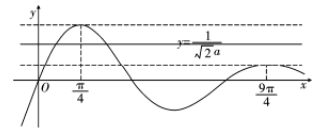【答案】 $a \in\left(\mathrm{e}^{\frac{\pi}{4}}, \mathrm{e}^{\frac{9 \pi}{4}}\right]$.

【解析】 原题$\Leftrightarrow f^{\prime}(x)=-\sqrt{2} a \sin x+\mathrm{e}^x=0$ 在 $(0,+\infty)$ 上有 2 个不同的实根 (变号) $\Leftrightarrow g(x)=\sin x \cdot \mathrm{e}^{-x}$ 的